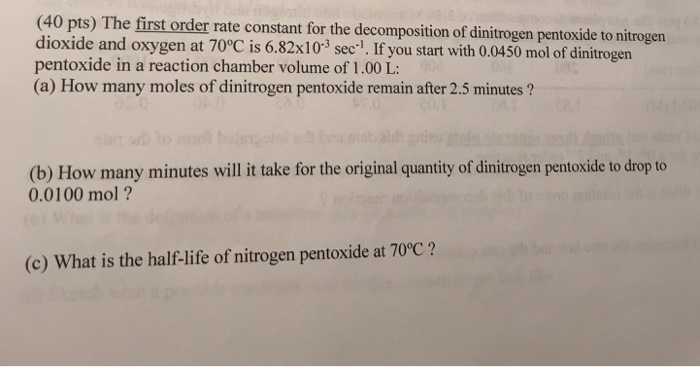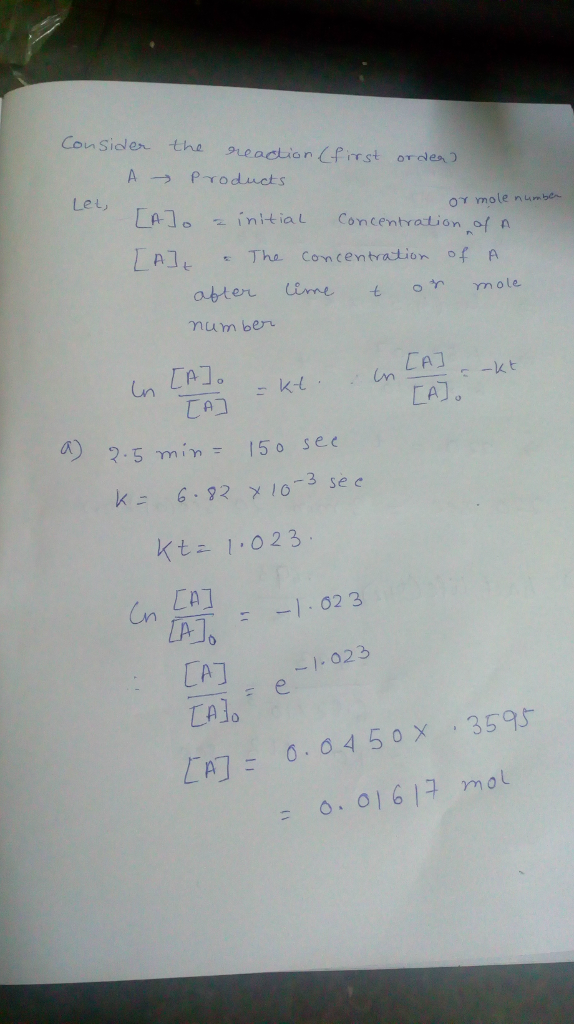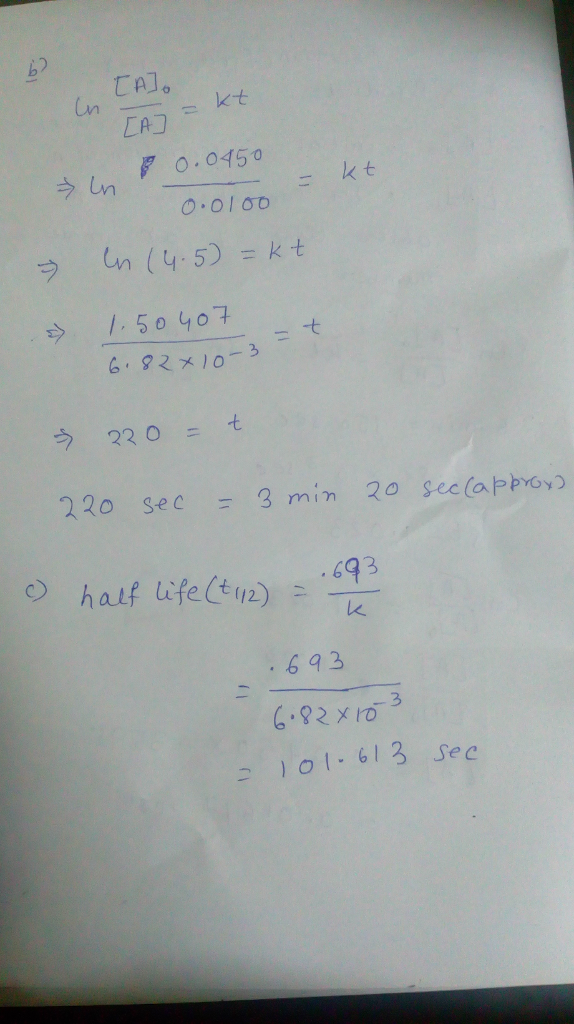# Question & Answer: The first order rate constant for the decomposition of dinitrogen pentoxide to nitrogen dioxide…..The first order rate constant for the decomposition of dinitrogen pentoxide to nitrogen dioxide and oxygen at 70 degree C is 6.82 times 10^-3 sec^-1. If you start with 0.0450 mol of dinitrogen pentoxide in a reaction chamber volume of 1.00 L: (a) How many moles of dinitrogen pentoxide remain after 2.5 minutes ? (b) How many minutes will it take for the original quantity of dinitrogen pentoxide to drop to 0.0100 mol? (c) What is the half-life of nitrogen pentoxide at 70 degree C?